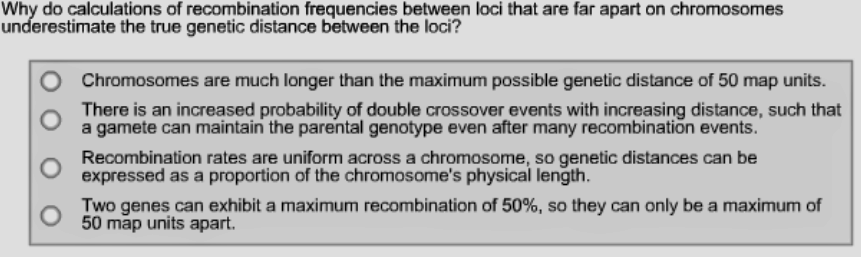# Problem: Why do calculations of recombination frequencies between loci that are far apart on chromosomes underestimate the true genetic distance between the loci?A. Chromosomes are much longer than the maximum possible genetic distance of 50 map units.B. There is an increased probability of double crossover events with increasing distance, such that a gamete can maintain the parental genotype after many recombination events.C. Recombination rates are uniform across a chromosome, so genetic distances can be expressed as a proportion of the chromosome's physical length.D. Two genes can exhibit a maximum recombination of 50%, so they can only be a maximum of 50 map units apart.

###### FREE Expert Solution

Following Sturtevant's observation regarding the three genes of Drosophila, it can be seen that recombination frequencies are significant contributors to the construction of a genetic map. In this map, the distances between genes are expressed in map units, with one map unit equivalent to a 1% recombination frequency. Therefore, the farther apart 2 genes are from one another, the larger the map unit value, as well as the recombination frequency.###### Problem Details

Why do calculations of recombination frequencies between loci that are far apart on chromosomes underestimate the true genetic distance between the loci?

A. Chromosomes are much longer than the maximum possible genetic distance of 50 map units.

B. There is an increased probability of double crossover events with increasing distance, such that a gamete can maintain the parental genotype after many recombination events.

C. Recombination rates are uniform across a chromosome, so genetic distances can be expressed as a proportion of the chromosome's physical length.

D. Two genes can exhibit a maximum recombination of 50%, so they can only be a maximum of 50 map units apart.